# Logarithmic residue

(diff) ← Older revision | Latest revision (diff) | Newer revision → (diff)

of a meromorphic function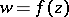at a pointof the extended complex-plane

The residueof the logarithmic derivative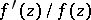at the point. Representing the functionin a neighbourhood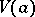of a pointin the form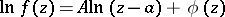, whereis a regular function in, one obtainsThe corresponding formulas for the case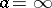have the form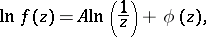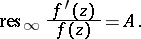Ifis a zero or a pole ofof multiplicity, then the logarithmic residue ofatis equal toor, respectively; at all other points the logarithmic residue is zero.

Ifis a meromorphic function in a domainandis a rectifiable Jordan curve situated inand not passing through the zeros or poles of, then the logarithmic residue ofwith respect to the contouris the integral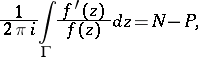(1)

whereis the number of zeros andis the number of poles ofinside(taking account of multiplicity). The geometrical meaning of (1) is that asis traversed in the positive sense, the vectorperformsrotations about the origin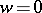of the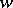-plane (see Argument, principle of the). In particular, ifis regular in, that is,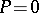, then from (1) one obtains a formula for the calculation of the index of the pointwith respect to the imageofby means of the logarithmic residue:(2)

Formula (2) leads to a generalization of the concept of a logarithmic residue to regular functions of several complex variables in a domainof the complex space,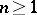. Let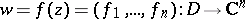be a holomorphic mapping such that the Jacobian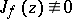and the set of zerosis isolated in. Then for any domain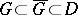bounded by a simple closed surfacenot passing through the zeros ofone has a formula for the index of the pointwith respect to the image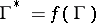: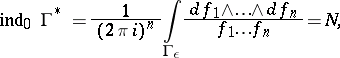(3)

where the integration is carried out with respect to the-dimensional framewith sufficiently small. The integral in (3) also expresses the sum of the multiplicities of the zeros ofin(see ).

How to Cite This Entry:
Logarithmic residue. Encyclopedia of Mathematics. URL: http://encyclopediaofmath.org/index.php?title=Logarithmic_residue&oldid=13475
This article was adapted from an original article by E.D. Solomentsev (originator), which appeared in Encyclopedia of Mathematics - ISBN 1402006098. See original article